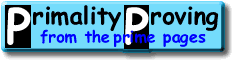Finding primes & proving primality 1: IntroductionHome > Primality Proving > Chapter One: Introduction

#### Introduction

How do we go about finding primes?  And once we have found them, how do we prove they are truly prime?  The answer depends on the size of the primes and how sure we need to be of their primality.  In these pages we present the appropriate answers in several sections.  Let us preview these chapters one at a time.

#### Chapter Two: The quick tests for small numbers and probable primes

For very small primes we can use the Sieve of Eratosthenes or trial division.  These methods are sure, and are the best methods for small numbers, but become far too time consuming before our numbers reach thirty digits.

If we are going to use our primes for "industrial" uses (e.g., for RSA encryption) we often do not need to prove they are prime.  It may be enough to know that the probability they are composite is less than 0.000000000000000000000001%.  In this case we can use (strong) probable primality tests.

These probable primality tests can be combined to create a very quick algorithm for proving primality for integers less than 340,000,000,000,000.

#### Chapter Three: The classical tests

A quick look at the list of largest known primes shows numbers with hundreds of thousands (even millions) of digits--and these are all proven primes (not probable primes)!  So how can we know they are prime?  Look at a portion of this list and decide what the numbers all have in common.
```  111  189*2^34233-1                  10308 Z  89
112  15*2^34224+1                   10304 D  93
113  (5452545+10^5153)*10^5147+1    10301 D  90 Palindrome
114  23801#+1                       10273 C  93 primorial plus one
115  63*2^34074+1                   10260 Y  95
116  213819*2^33869+1               10201 Y  93
```

They are all trivial to factor if we either add, or subtract, one!  This is no accident.

It is possible to turn the probable-primality tests of chapter two for an integer n into primality proofs, if we know enough factors of either n+1 and/or n-1.  These proofs are called the classical tests and we survey them in our third chapter.

These tests have been used for over 99.99% of the largest known primes.  They include special cases such as the Lucas-Lehmer test for Mersenne primes and Pepin's Test for Fermat primes.

#### Chapter Four: The General Purpose Tests

Finally, the obvious problem with the classical tests is that they depend on factorization--and it appears factoring is much harder than primality proving for the "average" integer.  In fact this is the key assumption behind the popular RSA encryption method!

Using complicated modern techniques, the classical tests have been improved into tests for general numbers that require no factoring such as the APR, APRT-CL and the ECPP algorithms.  In chapter four we say a few words about these methods, discuss which of these test to use (classical, general purpose...), and then leave you with a few references with which to pursue these tests.

In 2002 a long standing question was answered: can integers be proven prime in "polynomial time" (that is, with time bounded by a polynomial evaluated at the number of digits).  Some of the previous algorithms come close (ECPP is almost always polynomial, and is conjectured to always be polynomial bounded).  Agrawal, Kayal and Saxena answered this question in the affirmative by giving a "simple" polynomial time algorithm.  We present this algorithm in chapter four.

 [ next page | contents ] Copyright © 2023 PrimePages (Originally written by Chris Caldwell)